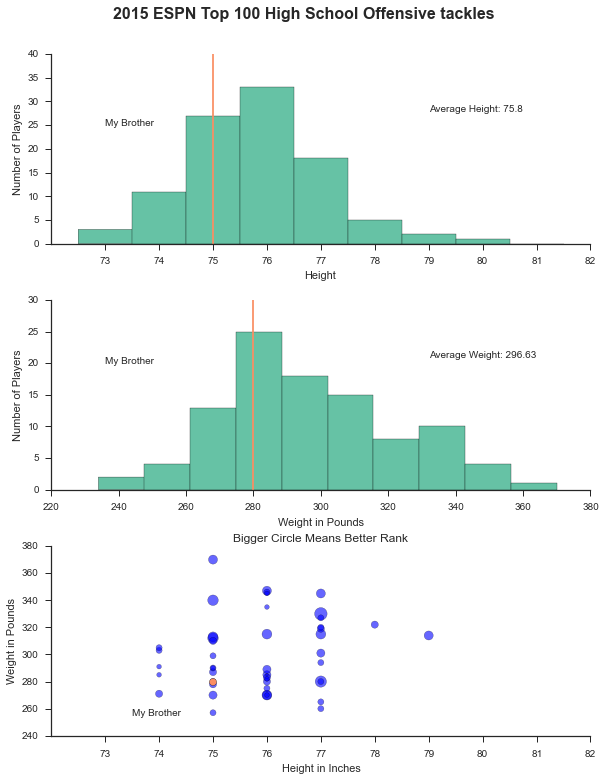# Offensive Tackles in Football¶

So I have a brother who is currently 16 and plays high school football as an offensive tackle. He is 6 feet 2 inches and weights 250 pounds. Now that is a big kid. His dream is to play professional football one day.

The first step to playing professional football is to play college football for hopefully a good team. Not knowing much about what it takes to get recruited by a top college football team, I thought I would look for some data. Fortunately, ESPN has height and weight data on the top 100 offensive tackles being recruited out of high school. This little project will look at the height and weight of top recruited offensive tackles and how these values are associated with that player's rank.

# Get and Clean the Data¶

In :
from bs4 import BeautifulSoup
import urllib2
import pandas as pd
from pandas import DataFrame, Series
%matplotlib inline
from __future__ import division
from matplotlib import pyplot as plt
import seaborn as sns
sns.set(style='ticks', palette='Set2')
import statsmodels.api as sm


Lets get the data from ESPN.

In :
html = urllib2.urlopen('http://espn.go.com/college-sports/football/recruiting/playerrankings/_/view/position/order/true/position/offensive-guard')
soup = BeautifulSoup(text.replace('ISO-8859-1', 'utf-8'))

In :
ht_wgt = []
for tr in soup.findAll('tr')[1:]:
tds = tr.findAll('td')
height = tds.text
weight = tds.text


A quick sanity check to make sure we got 100 players

In :
#should have 100
len(ht_wgt)

Out:
100

Now lets drop our data into a Pandas data frame and take a look.

In :
data = DataFrame(ht_wgt, columns=['height', 'weight', 'grade'])

Out:
0 6'5'' 330 87
1 6'5'' 280 85
2 6'3'' 340 84
3 6'3'' 312 84
4 6'5'' 315 83

Lets clean up the data to get the values as integers and convert the height to inches. I also created a mean zero grade just to bring the grades closer to zero.

In :
data['weight'] = data.weight.astype(int)
hgt_str = data.height.values
hgt_str = [x.split("'") for x in hgt_str]
hgt_in = [(int(x) * 12) + int(x) for x in hgt_str]
data['height_inches'] = hgt_in

Out:
0 6'5'' 330 87 77 10.48
1 6'5'' 280 85 77 8.48
2 6'3'' 340 84 75 7.48
3 6'3'' 312 84 75 7.48
4 6'5'' 315 83 77 6.48

# Make Some Plots¶

Lets take a look at the distribution of height and weight and how that fits in with the ranking. Also, I will plot my brother on the plots to see how he stacks up.

In :
fig, ax = plt.subplots(3,1)
fig.set_size_inches(8.5, 11)

fig.suptitle('2015 ESPN Top 100 High School Offensive tackles',
fontsize=16, fontweight='bold')

ax.hist(data.height_inches, bins = range(73,83), align='left')
ax.set_xlabel('Height')
ax.set_ylabel('Number of Players')
ax.annotate('Average Height: {}'.format(data.height_inches.mean()),
xy=(.5, .5), xytext=(.70, .7),
xycoords='axes fraction', textcoords='axes fraction')
ax.plot([75, 75], [0,40])
ax.set_xlim([72,82])
ax.set_xticks(range(73,83))
ax.annotate('My Brother', xy=(75, 20), xytext=(73, 25))

ax.hist(data.weight)
ax.set_xlabel('Weight in Pounds')
ax.set_ylabel('Number of Players')
ax.annotate('Average Weight: {}'.format(data.weight.mean()),
xy=(.5, .5), xytext=(.70, .7),
xycoords='axes fraction', textcoords='axes fraction')
ax.plot([280, 280], [0,30])
ax.annotate('My Brother', xy=(250, 15), xytext=(236, 20))

ax.scatter(data.height_inches, data.weight, s=data.grade_meanzero*15, alpha=.6)
ax.set_title('Bigger Circle Means Better Rank')
ax.set_xlabel('Height in Inches')
ax.set_ylabel('Weight in Pounds')
ax.set_xlim([72,82])
ax.set_xticks(range(73,83))
ax.scatter(,, alpha=1, s=50, c=sns.color_palette("Set2", 2))
ax.annotate('My Brother', xy=(75, 280), xytext=(73.5, 255))

fig.tight_layout()